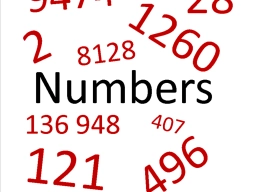# Twice 2

Twice the difference between a number and 3 is equal to three times the sum of the number and 8. Find the number.

x =  -30

### Step-by-step explanation:Did you find an error or inaccuracy? Feel free to write us. Thank you!

Tips for related online calculators
Do you have a linear equation or system of equations and looking for its solution? Or do you have a quadratic equation?